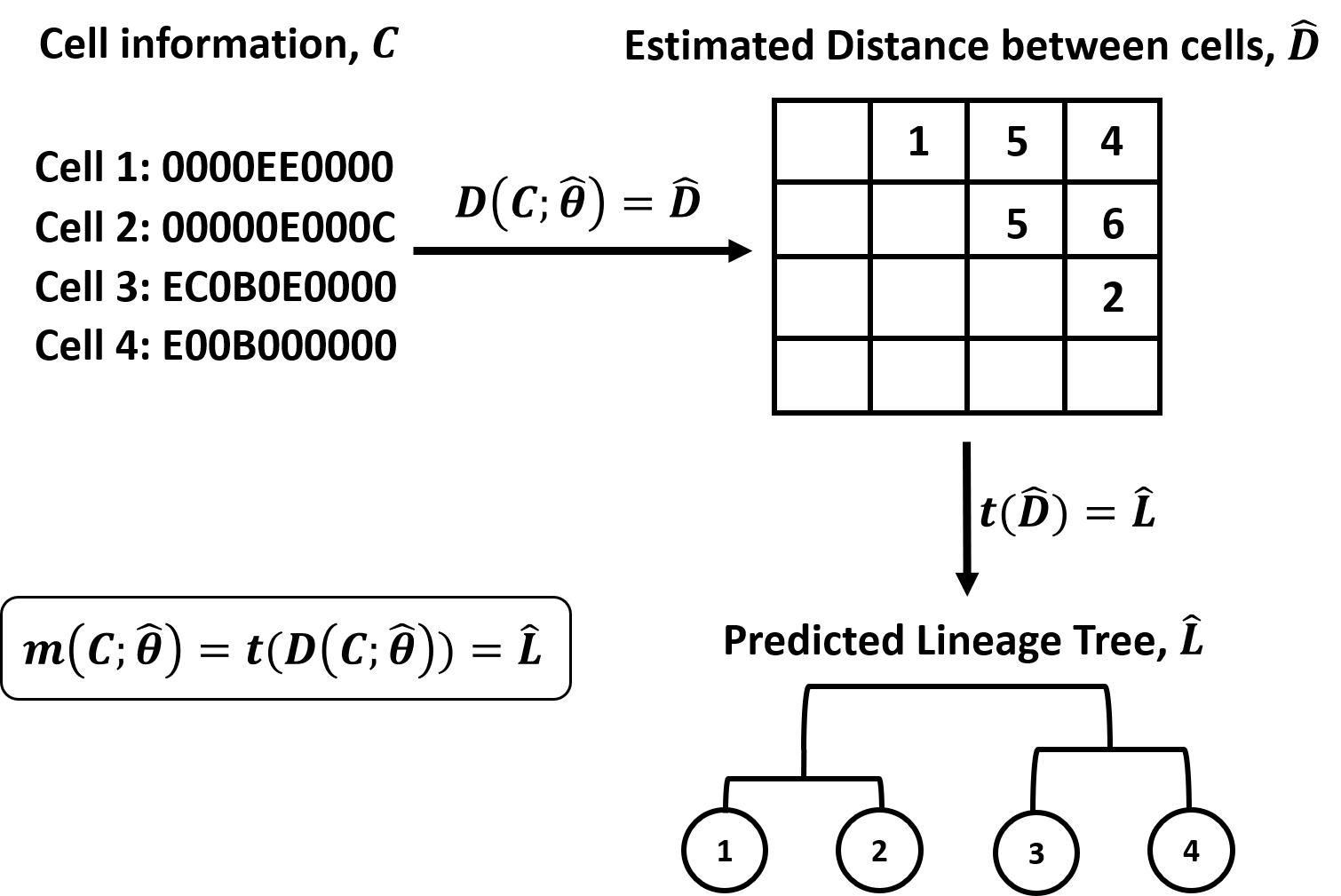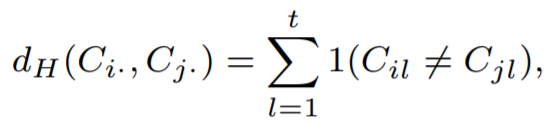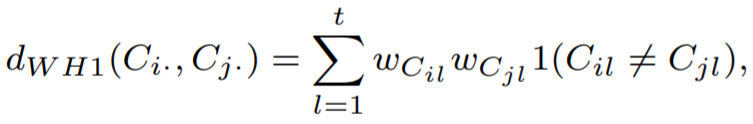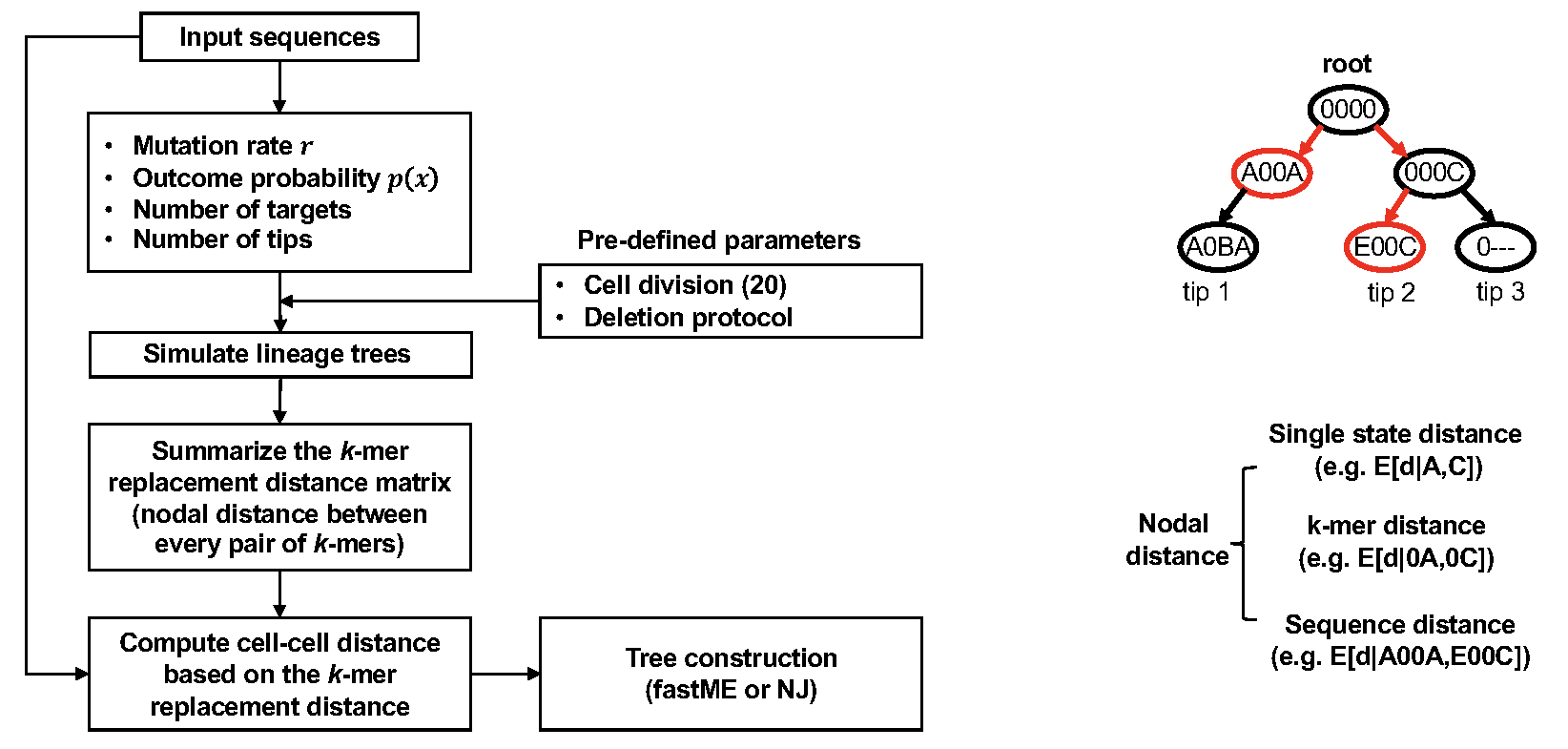# Distance based Cell LinEAge Reconstruction (DCLEAR)

Il-Youp Kwak (ikwak2@cau.ac.kr) and Wuming Gong (gongx030@umn.edu)

R/DCLEAR is an R package for Distance based Cell LinEAge Reconstruction(DCLEAR). These codes are created during the participation of Cell Lineage Reconstruction DREAM challenge.

## DCLEAR Overview

Figure 1. Overview of DCLEAR modeling architecture. Our model is divided into two parts, 1) estimating distance between cells and 2) constructing tree using distance matrix.### Estimating distance between cells

Naive approach would be the hamming distance that simply calculate the edit distance.However, the previous approach assume every base difference have same weights. For example, two sequences, ‘00AB0’ and ‘0-CB0’, are different at second and third positions. The second position, we have ‘0’ and ‘-’, and the third position, we have ‘A’ and ‘C’.

For ‘0’ and ‘-’, ‘-’ is point missing and it is possibly ‘0’. Thus it should have lower weight. For ‘A’ and ‘C’, During the cell propagation, ‘0’ differentiated to ‘A’ and ‘0’ differentiated to ‘C’. Thus it should have larger weight. We can assign weights as below equation.And we can approximate unknown weights using training data.

### K-mer replacement distanceDCLEAR also implements a k-mer replacement distance (KRD), which does not require training data. KRD method first looks at mutations in the character arrays to estimate the parameters of the generative process associated with the tree to be reconstructed. With these parameters, we repetitively simulated trees with a size and mutation distribution similar to the target tree. The k-mer replacement distances were estimated from the simulated lineage trees and used to compute the distances between input sequences in the character arrays of internal nodes and tips. Specifically, by examining the simulated lineage trees, KRD estimated the expected 1-mer replacement distance between characters in the array (including ground state “0” and deletion state “-“) in the lineage trees and the probability for a given nodal distance of replacing a character in a cell array. To extend the 1-mer replacement distance to the k-mer replacement distance, the posterior probability distributions of k-mer replacement distance were estimated by using a conditional model considering a dependance for the concurrence of mutations. We found that by considering the neighboring characters, the conditional model can more accurately estimate the nodal distance than an independent model. The cell distance can then be readily computed as the mean expected k-mer replacement distance.

### Constructing tree from the distance matrix

With the previously proposed distance matric, we can construct distance matrix among cells. We can apply tree construction algorithms such as Neighbor-Joining(NJ), FastME.

## Usage

• How to use weighted hamming : Link
• How to use kmer_replacement : Colab Link
• Preparation for subchallenge 2 submission : Colab Link
• Preparation for subchallenge 3 submission : link

## installation

With ‘devtools’:

``devtools::install_github("ikwak2/DCLEAR")``